Continued from part 1 from 22.12.98 on this server
by W.D. Bauer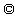released 20.1.99

This is done here by a library C-routine from  or by programs like MATHCAD, MAPLE or MATHEMATICA.
Fig 1a) and 1b) shows the asymmetric electric field inputs E(t) dependent from time t. We solved numerically two cases for each pulse shape: very good heat conduction (q=1,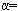10) and poor heat conduction (q=1,0.1). The results are shown in the fig. 2a),b)+c) and fig. 3a),b)+c) respectively. The a) diagrams show the solution T(t). The b) +c) diagrams show the T-S diagram for the pulse form indicated in the figure text.
The main result of the model calculation is that, for a unique dielectrics, it is irrelevant which pulse form is applied. The orientation of the path of an unique dielectrics in the T-S diagram shows always thermal losses for all calculated examples and allows no overunity result of the cycle. Therefore, my initial suggest that a ratched voltage form could transport heat into the dielectrics is wrong. The main error was that the suggest that a ferroelectrics get hot under a field. The adiabate equation shows the reverse. If a field is applied a ferroelectrics gets cool, a paraelectrics gets hot. Under discharge, a ferroelectrics get hot, a paraelectrics gets cool.

Nevertheless, there exist physical experiments on non-unique dielectrics which make probable that there can exist cycles in the D-E diagram of dielectrics with clockwise orientation which is equivalent to an overunity cycle as shown in . This can be read off from published measurements on polycristalline barium titanate dielectric ceramics by Partington et al. whose results are confirmed in parts by Koch [4,5] with respect to polycristalline ferroelectric behavior of barium titanates.
However, much more interesting for us is the paraelectric state, which was not examined by Koch. Partington et al. investigated a self baked mixed barium-strontium-titanate ceramics as dielectrics at temperatures where the substance was in the paraelectric state (left to Curie point (50 degree C) at probably 70 degree C). They measured the differential AC-permittivityof the material which is represented bywhich is the slope of characteristic D-E diagram of the capacitance. The principal electrical test setup is shown in fig.4. Due to internal relaxation of the crystal the material slowly shifted its permittivity values under field influence of 3300V to lower permittivity values. After switching off the field the permittivity values jumped again to higher which -however were lower before the material was charged and relaxed againwith time to the higher initial values. All these features are shown in fig.5. The nearer the temperature to the Curie point the bigger were the effects. Similary, the higher the the field the clearer the effect. In fig.6a) and fig.6b) these results from fig.5 are represented as slopesin the D-E diagram of the dielectrics in order to make conclusions on the qualitative behavior of the dielectric. Under zero voltage the positition and direction of the D-E-lines can be concluded clearly from the data in fig.5. For higher voltages two possibilities exist. The first is represented in fig.6a) and reproduces the qualitative shape of the D-E-line of a paraelectric material. However, it represents a negative overunity hysteresis area and would confirm very well a prediction made in a prior article . The other possibilities shown in fig.6b. Here the quite unsual paraelectric D-E-discharge line of the capacitance can cross the D-E- charging line one or more times. In this case no prediction can be made by the author because under this conditions positive and negatively oriented working areas appear in the closed working area of a cycle of the dielectrics and allow no prediction at first hand.
Therefore, the standard thermodynamic description of the problem is not possible because the effects are due to time-dependent varying material parameters. Standard thermodynamic would need unique material properties independent from time.
Therefore, whether it is possible to rebuild and improve such materials is a task for a material scientist now and can not be followed here by me here.

Bibliography:

1) G. Engeln-Müllges, F.Reutter
Formelsammlung zur numerischen Mathematik mit C-Programmen
B.I. Wissenschaftsverlag Mannheim,Wien,Zürich 1990

3)J.R.Partington, G.V. Planer, I.I.Boswell
Philos.Mag., Ser.7, 40,(1949)157-175

4)H.J. Martin
Die Ferroelektrika

5) W.Koch, Z.Naturforschg. 13 a, p.303-310
Ferroelekrische Nachwirkungerscheinungen an polykristallinem Bariumtitanat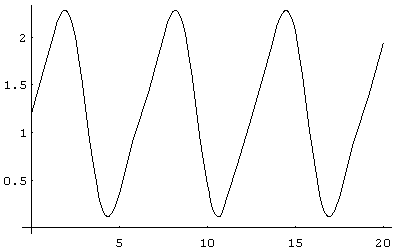fig.1a: The electrical input signal E(t)=1.2+sin(x)-.22sin(2x)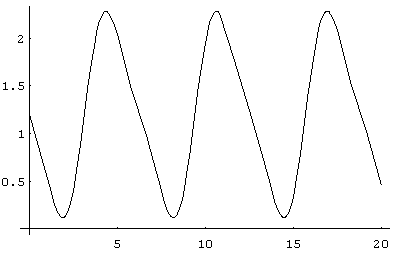fig.1b: The electrical input signal E(x)=1.2-sin(x)+.22sin(2x)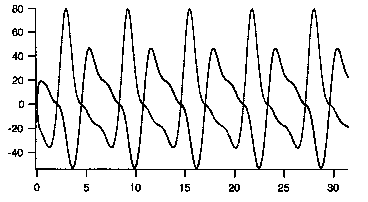fig.2a:Temperature T versus time t (for q=110)
upper trace at the starting point: signal from fig. 1b)
lower trace at the starting point: signal from fig.1a)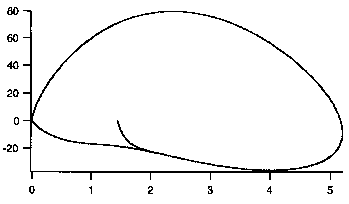fig.2b: limit cycle temperature T versus E2, i.e. entropy S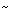E2 for E(t) from fig.1a) and q=1,10fig.2c: limit temperature T versus E2, i.e. entropy SE2 for E(t) from fig.1b) and q=1,10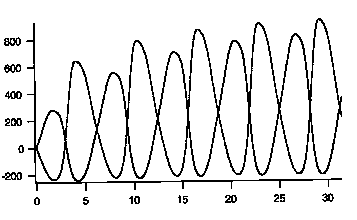fig.3a:Temperature T versus time t (for q=10.1)
upper trace at the starting point: signal from fig. 1b)
lower trace at the starting point: signal from fig.1a)fig.3b: limit cycle temperature T versus E2, i.e. entropy SE2 for E(t) from fig.1a) and q=1,0.1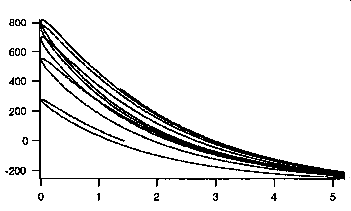fig.3c: limit temperature T versus E2, i.e. entropy SE2 for E(t) from fig.1b) and q=1,0.1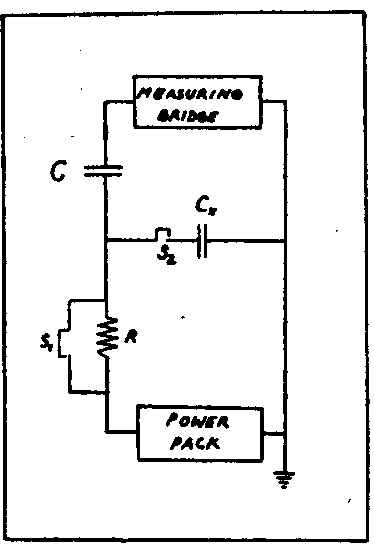fig.4: the principal setup for AC measurement acc. to Partington, Planer and Boswell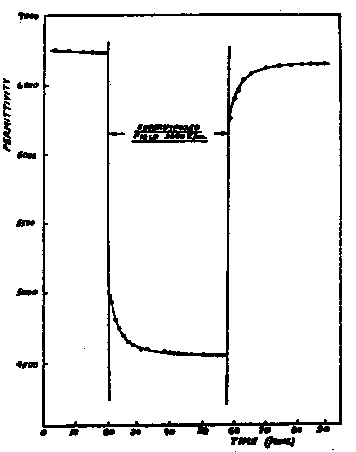fig.5: the dielectric permittivity relaxation of barium-strontium-titanate ceramics above the Curie-point (70 degree C ??)
abcissa:time in 10 minutes per tick, ordinate: permittivity; voltage applied in the time window from 20 to 60 min.:3300V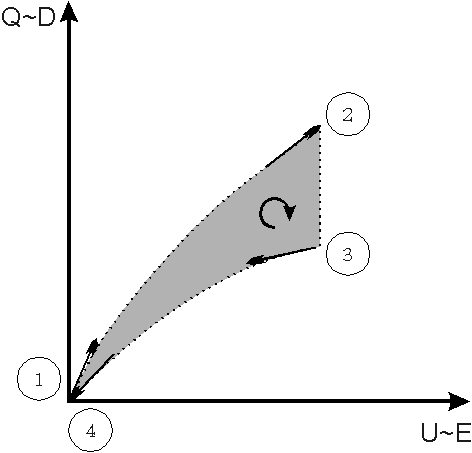fig.6a: tentative reconstruction of the D-E-diagram from the measurement values from fig.5
1.possibility: negative overunity hysteresis, typical D-E-line of the paraelectric material
slope 1:before switching on the field at t=20min; slope 2:shortly after switching on the field at t=20min; slope 3:after relaxation in field shortly before switching off the field at t=60min; slope 4:shortly after switching off the field at t=60min;fig.6b: tentative reconstruction of the D-E-diagram from the measurement values from fig.5
2.possibility: two (or more) working areas of positive and negative orientation, untypical D-E-line of paraelectrical material, no clear prediction regarding efficiency
slope 1:before switching on the field; slope 2:shortly after switching on the field; slope 3:after relaxation iin field shortly before switching off the field; slope 4:shortly after switching off the field;

back to the theory page